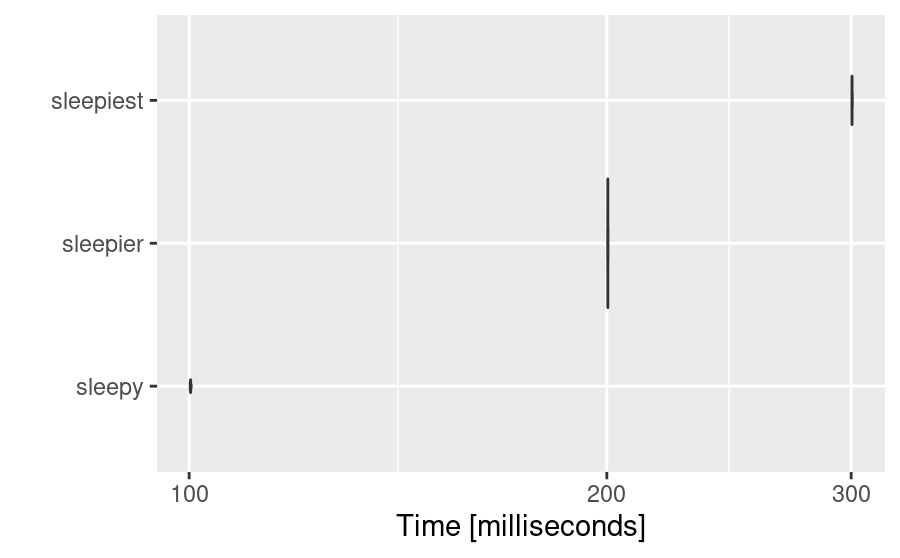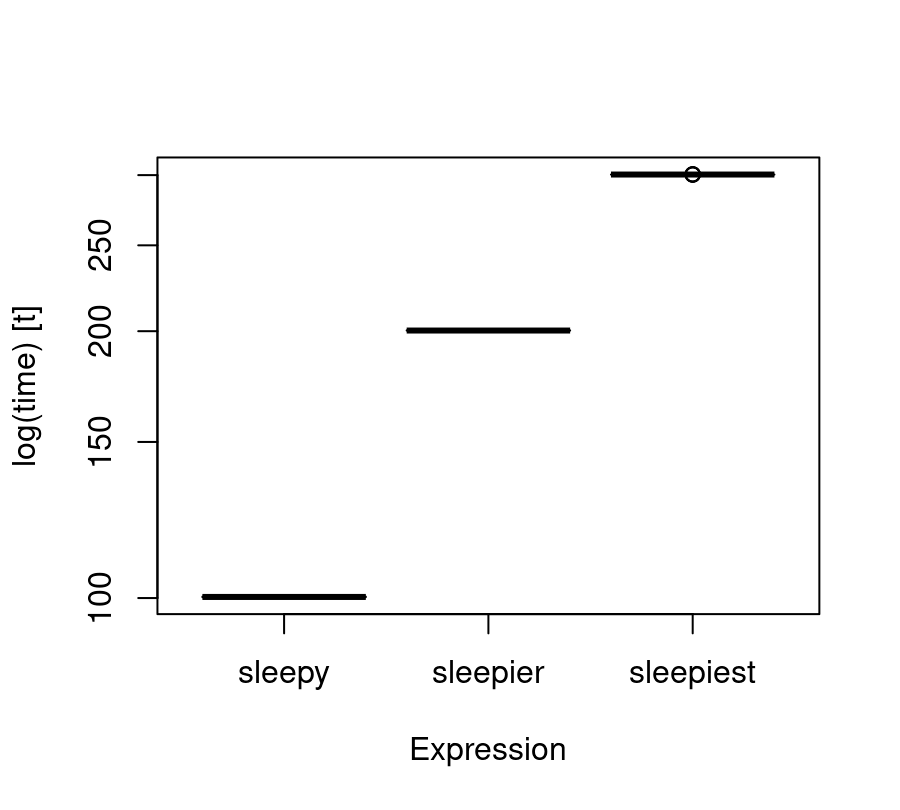GitHub

# Timing in R

Published: November 20, 2017As time goes on, your R scripts are probably getting longer and more complicated, right? Timing parts of your script could save you precious time when re-running code over and over again. Today I’m going to go through the 4 main functions for doing so.

## Nested timings

### 1) `Sys.time()`

`Sys.time()` takes a “snap-shot” of the current time and so it can be used to record start and end times of code.

``````start_time = Sys.time()
Sys.sleep(0.5)
end_time = Sys.time()
``````

To calculate the difference, we just use a simple subtraction

``````end_time - start_time
## Time difference of 0.5027 secs
``````

Notice it creates a neat little message for the time difference.

### 2) The {tictoc} package

You can install the `CRAN` version of {tictoc} via

``````install.packages("tictoc")
``````

whilst the most recent development is available via the {tictoc} GitHub page.

``````library("tictoc")
``````

Like `Sys.time()`, {tictoc} also gives us ability to nest timings within code. However, we now have some more options to customise our timing. At it’s most basic it acts like `Sys.time()`:

``````tic()
Sys.sleep(0.5)
toc()
## 0.505 sec elapsed
``````

Now for a more contrived example.

``````# start timer for the entire section, notice we can name sections of code
tic("total time")
# start timer for first subsection
tic("Start time til half way")
Sys.sleep(2)
# end timer for the first subsection, log = TRUE tells toc to give us a message
toc(log = TRUE)
## Start time til half way: 2.013 sec elapsed
``````

Now to start the timer for the second subsection

``````tic("Half way til end")
Sys.sleep(2)
# end timer for second subsection
toc(log = TRUE)
## Half way til end: 2.005 sec elapsed
# end timer for entire section
toc(log = TRUE)
## total time: 4.027 sec elapsed
``````

We can view the results as a list (`format = TRUE` returns this list in a nice format), rather than raw code

``````tic.log(format = TRUE)
##    user  system elapsed
##   0.000   0.000   1.001
``````

We only want to take notice of the “elapsed” time, for the definition of the “user” and “system” times see this thread.

For a repeated timing, we would use the `replicate()` function.

``````system.time(replicate(10, Sys.sleep(0.1)))
##    user  system elapsed
##   0.004   0.000   1.004
``````

### 2) The microbenchmark package

You can install the `CRAN` version of {microbenchmark} via

``````install.packages("microbenchmark")
``````

Alternatively you can install the latest update via the {microbenchmark} GitHub page.

``````library("microbenchmark")
``````

At it’s most basic, `microbenchmark()` can we used to time single pieces of code.

``````# times = 10: repeat the test 10 times
# unit = "s": output in seconds
microbenchmark(Sys.sleep(0.1), times = 10, unit = "s")
## Unit: seconds
##            expr    min     lq   mean median     uq    max neval
##  Sys.sleep(0.1) 0.1001 0.1002 0.1002 0.1002 0.1002 0.1002    10
``````

Notice we get a nicely formatted table of summary statistics. We can record our times in anything from seconds to nanoseconds(!!!!). Already this is better than `system.time()`. Not only that, but we can compare sections of code in an easy-to-do way and name the sections of code for an easy-to-read output.

``````sleep = microbenchmark(sleepy = Sys.sleep(0.1),
sleepier = Sys.sleep(0.2),
sleepiest = Sys.sleep(0.3),
times = 10,
unit = "s")
``````

As well as this (more?!) {microbenchmark} comes with a two built-in plotting functions.

``````microbenchmark:::autoplot.microbenchmark(sleep)
````````````microbenchmark:::boxplot.microbenchmark(sleep)
``````These provide quick and efficient ways of visualising our timings.

## Conclusion

`Sys.time()` and `system.time()` have there place, but for most cases we can do better. The {tictoc} and {microbenchmark} packages are particularly useful and make it easy to store timings for later use, and the range of options for both packages stretch far past the options for `Sys.time()` and `system.time()`. The built-in plotting functions are handy.

Thanks for chatting!